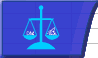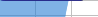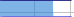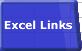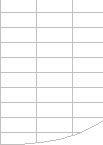# Array FormulaeImportance of Speed Faster Calculations Microsoft's Advice Bottlenecks/Size Lookups Dynamic Ranges Totalling Generating Workbooks Multi-Level Calculations Array Formulae SUMIF Example Worksheet Links## Array Formulae

Array formulae are one of Excel’s most powerful features, although not always the easiest to use. Look at Bob Umlas's excellent paper at http://www.emailoffice.com/excel/arrays-bobumlas.html for many examples of Array formulae, and also Chip Pearson's page at http://www.cpearson.com/excel/array.htm .

Single-cell array formulae are evaluated multiple times, depending on the number of cells referred to in the formula. This can take significant time, and may or may not be faster than the alternatives.
A range array formula covering multiple cells may be faster to calculate than individual formulae in each cell (although the speed advantage seems less in Excel97 and Excel2000).
Because array formulae and functions like SUM which reference ranges influence the sequence in which Excel calculates, you should try and avoid mixing row and column references or overlapping array references.

#### How Microsoft Excel Expands Array Formulas

When you use an array constant in a formula or function, the other components or arguments should have the same dimensions as the first array. If necessary, Microsoft Excel expands the necessary components to the required dimensions. Each component must have the same number of rows as the component with the greatest number of rows, and the same number of columns as the component with the greatest number of columns.

For example, in the formula =SUM({ 1,2,3 }*4), one component is a l-by-3 array and the other is a single value. In evaluating this formula, Microsoft Excel automatically expands the second component to a l-by-3 array and evaluates the formula as =SUM({ 1,2,3}*{4,4,4}). The formula's result equals 24, which is the sum of 1*4, 2*4, and 3*4.

The following table shows how array components or arguments are expanded.

 In this formula The arguments are evaluated as Explanation =1+{1,2,;3,4} {1,1;1,1}+{1,2;3,4} The constant 1 is expanded to match the array. ={1,2,3}*{2;3} {1,2,3;1,2,3}* {2,2,2;3,3,3} Each array is expanded to accommodate the dimensions of the other. ={1,2}+{1,2,3;4,5,6} {1,2,#N/A;1,2,#N/A}+ {1,2,3;4,5,6} Each array is expanded, but no value exists in the first array to add to the third column values in the second array. =MOD({5,6,7},4) MOD({5,6,7},{4,4,4}) The constant 4 is expanded to match the array

You should enter an array formula in a range of cells with the same dimensions as the resulting array produced by the formula. Microsoft Excel can then place each value in the resulting array into one cell of the array range.

• If an array formula produces an array smaller than the selected array range, Microsoft Excel expands the resulting array to fill the range.
• If Microsoft Excel expands an array to fill a range larger than the array formula, #N/A error values appear in cells for which no valid expandable value is available.
• If an array formula produces an array larger than the selected array range, the excess values do not appear on the worksheet.

For example, the formula ={ 1,2,3}*{2,3,4} produces the l-by-3 array {2,6,12}.

• If you enter this formula into a 2-by-3 array range, Microsoft Excel expands the result to {2,6,12;2,6,12}.
• If you enter the same formula into a l-by-4 array range, Microsoft Excel expands the result to {2,6,12,#N/A}.
• If you enter the same formula into a l-by-2 array range, the result is {2,6}.

Microsoft Excel includes some worksheet functions that return arrays of values or require either array constants or references to cell ranges as arguments.

For example, you want to compute the trend of your monthly sales over the last six months. That trend is described not by one value, but by sixthe trend values corresponding to the sales in each of the last six months. Microsoft Excel provides a worksheet function, TREND, that performs this computation for you and produces the six values. You enter the trend formula into 6 adjacent cells using Control/Shift/Enter.

The Microsoft Excel functions that work with arrays are included in the following table.

 Function Name Array usage COLUMN Returns an array when the argument is a range COLUMNS Requires an array or cell range as an argument GROWTH Requires arrays or cell ranges as arguments and can return an array HLOOKUP Requires an array or cell range as an argument INDEX (array form) Requires an array as an argument and can return an array LINEST Always returns an array LOGEST Always returns an array LOOKUP (array form) Requires an array or cell range as an argument MATCH Requires an array or cell range as an argument MDETERM Requires an array as an argument MINVERSE Always returns an array MMULT Always returns an array ROW Returns an array when the argument is a range ROWS Requires an array or cell range as an argument SUMPRODUCT Requires arrays, cell ranges, or values as arguments TRANSPOSE Always returns an array TREND Requires arrays or cell ranges as arguments and can return an array VLOOKUP Requires an array or cell range as an argument

There are also other functions that can take either single-value arguments or array arguments. These functions return different results when used with array arguments. For more information about functions, click the Function Wizard button on the Standard toolbar or see online Help.

Caution

Before you enter a function on a worksheet, you need to know what the function returns. For example, the MINVERSE (matrix inverse) function returns an array the same size as its argument. Another function, TRANSPOSE, returns an array with the opposite dimensions of its argument. Make sure you allow space on your worksheet for the resulting array.

#### Minimise the Number of Array Operations

 Minimise the number of cells and expressions evaluated. Use helper columns or rows to take as many cell references as possible out of the array formulae The main key to optimising the calculation speed of Array formulae is to make sure that the number of cells and expressions evaluated in the array formula is as small as possible. Remember that an array formula is a bit like a volatile formula: if any single one of the cells it references has changed or is volatile or has been recalculated then the array formula will evaluate ALL the cells it references. Take expressions and range references out of the array formulae into separate helper columns/rows. This will make much better use of Excel's smart recalculation process. Don’t reference complete rows, or more rows and columns than you need. Use Dynamic Range Names  where possible. Even though they are volatile its worthwhile because they minimise the size of the ranges. Be careful with array formulae that reference both a row and a column: this forces the calculation of a rectangular range. Use SUMPRODUCT if possible: it’s slightly faster than the equivalent array formula.

#### Array formulae SUM with multiple conditions

One frequent use of Array formulae is to do a sum with multiple conditions. This relatively easy to do, particularily if you use Excel's Conditional Sum Wizard, but often very slow. Usually there are alternative ways of getting the same result, but much faster.

Two good methods of speeding up multiple condition SUMs are:

• Split out the multiple conditions into a column of helper formulae that return True or False for each row, then reference the helper column in a SUMIF or array formulae. Whilst it may seem that for a single array formula that this does not reduce the number of calculations, in fact most of the time this allows Excel's smart recalculation process to only recalculate the formulae in the helper column that NEED to be recalculated.
• If the data can be sorted then a good technique is to count groups of rows and limit the array formulae to looking at the subset groups.

See SUMIF example for a worked example. The downloadable FastExcel Sample Problem also has an example which shows the method and the considerable time saving which is often achievable.

#### Using SUMPRODUCT for multiple condition array formulae.

There are some advantages to using SUMPRODUCT rather than SUM array formulae:

• SUMPRODUCT does not have to be array-entered using Control-shift-enter.
• SUMPRODUCT is usually slightly faster (5-10%).
• SUMPRODUCT can be made to treat blanks and text as zero.

You can use SUMPRODUCT for multiple condition array formulae as follows:

SUMPRODUCT(--(Condition1),--(Condition2),RangetoSum)

where Condition1 & 2 are conditional expressions such as \$A\$1:\$A\$10000<=\$Z4. Because conditional expresssions return True or False rather than numbers they need to be coerced to numbers inside the SUMPRODUCT function. You can do this using two minus signs (--) or by adding 0 (+0) or by multiplying by 1 (*1). Using -- is very slightly faster than +0 or *1.

Bug in Excel 2007 SP2, subsequently fixed in 12.0.6545.5000: If you use the 2 minus signs (--) syntax in an array formula or SUMPRODUCT formula with a direct reference to a volatile function in Excel 2007 SP2 the formula does not recalculate when the vloatile function referred to recalculates. (this works correctly in all other Excel versions). For Example:
A1:A5 =RAND()
B1:B5 {=-A1:A5}
Cells B1:B5 will not recalculate when you press F9. Thanks to Luke Wisbey for letting me know about this bug.

Note that the size and shape of the ranges or arrays used in the conditional expressions and range to sum must be the same, and cannot contain entire columns.

You can also directly multiply the terms inside SUMPRODUCT rather than separate them by commas:

SUMPRODUCT((Condition1)*(Condition2)*RangetoSum)

But this is usually slightly slower than using the comma syntax, and gives an error if the range to sum contains a text value.
However it is slightly more flexible in that the range to sum may have for instance multiple columns when the conditions only have 1 column.

#### Using SUMPRODUCT to multiply and add ranges and arrays.

In cases like weighted average calculations where you need to multiply a range of numbers by another range of numbers and sum the results using the comma syntax for SUMPRODUCT can be 20-25% faster than an array entered SUM:

{=SUM(\$D\$2:\$D\$10301*\$E\$2:\$E\$10301)}

=SUMPRODUCT(\$D\$2:\$D\$10301*\$E\$2:\$E\$10301)

=SUMPRODUCT(\$D\$2:\$D\$10301,\$E\$2:\$E\$10301)

These three formulae all produce the same result, but the third formula using the comma syntax for SUMPRODUCT only takes about 77% of the time to calculate that the other two formulae need.

#### Use DSUM instead of multiple condition array formulae.

If you only have a few array formulae sums with multiple conditions you may be able to use DSUM instead. DSUM is significantly faster than equivalent array formulae. The disadvantage of DSUM is that the criteria have to be in a separate range, which makes it impractical to use and maintain in many circumstances.

#### Use FastExcel AVLOOKUPS.

You can use the FastExcel Version 2 AVLOOKUPS function inside a SUM function to return multiple rows and SUM them. If you are doing multiple conditional sums, particularly on sorted data, AVLOOKUPS can be significantly faster than array formulae.

#### Array and Function Calculation Bottlenecks

Excel’s calculation engine is optimised to exploit array formulae and functions that reference ranges. However some unusual arrangements of these formulae and functions can sometimes, but not always, cause significantly increased calculation time.

If you find a calculation bottleneck involving array-formulae and range functions look for:

• Partially overlapping references:
• Array formulae/range functions which reference part of a block of cells that are calculated in another array formula/range function. This situation can frequently occur in time series analysis.
• One set of formulae referencing by row, and a second set of formulae referencing the first set by column:
• A large set of single-row array formulae covering a block of columns, with SUM functions at the foot of each column.

Plus points of Array Formulae:

• Array Formulae are concise: You can eliminate columns and rows by packing the calculations into an array formula.
• Array Formulae are powerful: You can easily perform many complex calculations such as multiple conditional sums and counts using array formulae.
• Array Formulae save disk space: Using array formulae covering a range of cells can reduce workbook size compared to equivalent individual formulae. Memory used does not usually seem to reduce significantly.
• Array Formulae offer increased protection: Excel will only allow you to alter a complete array formula block so the user is prevented from accidentally changing a single formula.

Minus points of Array Formulae:

• The Black-Box effect: Array Formulae can be complex and hard to understand. Most Excel users do not understand array formulae at all. This can reduce confidence and usability of your spreadsheet.
• Calculation Overhead: Each time an array formula is calculated all of the virtual cells needed by the array formula are calculated, regardless of whether this is required or not. This may cause the array formula to be slower than a non-array equivalent set of formulae.
• Requirement for all components of the array formulae to be the same size: This may require the array formula to perform a large number of unnecessary calculations. This problem frequently occurs in a SUM with multiple conditions problem. Sorting and then calculating the subset range that needs to be summed may often make significant calculation speed improvements. The SUMIF example shows how several hours of Array Calculations can be reduced to under a second.© 2001-2020 Decision Models Legal Disclaimer Privacy Statement